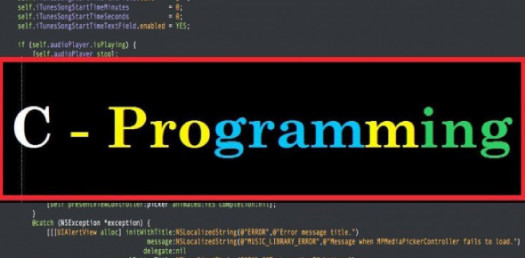# Hardest Trivia Quiz On Output Of C Programs! Test

10 Questions | Total Attempts: 281Settings.

Related Topics
• 1.
What will be output if you will compile and execute the following c code? #include int main(){   int a=sizeof(a);   a=modify(a);   printf("%d",a);   return 0; }What will be output if you will compile and execute the following c code? #include int main(){   int a=sizeof(a);   a=modify(a);   printf("%d",a);   return 0; } int modify(int x){   int y=3;   _AX=x+y;   return; }
• A.

2

• B.

3

• C.

5

• D.

Garbage Value

• E.

None of the above

• 2.
What will be the output of the following arithmetic expression ? 5+3*2%10-8*6
• A.

-37

• B.

-42

• C.

-32

• D.

-28

• 3.
What will be the output of the following statement ? printf( 3 + "goodbye");
• A.

Goodbye

• B.

Odbye

• C.

Dbye

• D.

Bye

• 4.
Void main() { int a=10,b=20; char x=1,y=0; if(a,b,x,y) { printf("EXAM"); } } What is the output?
• A.

XAM is printed

• B.

Exam is printed

• C.

Compiler Error

• D.

Nothing is printed

• 5.
What will be the output of the following statement ? printf("%X%x%ci%x",11,10,'s',12);
• A.

Error

• B.

Basc

• C.

Bas94c

• D.

None of these

• 6.
What will be the value of `a` after the following code is executed #define square(x) x*x a = square(2+3)
• A.

25

• B.

13

• C.

11

• D.

10

• 7.
What would be the output of the following program? #include main() { char str[]="S\065AB"; printf("\n%d", sizeof(str)); }
• A.

7

• B.

6

• C.

5

• D.

Error

• 8.
What will be the output of the following statements ? int i = 3; printf("%d%d",i,i++);
• A.

34

• B.

43

• C.

44

• D.

33

• 9.
What will be output if you will compile and execute the following c code? #include int main(){   float f;   f=3/2;   printf("%f",f);   return 0; }
• A.

1.5

• B.

1.500000

• C.

1.000000

• D.

Compiler error

• E.

None of above

• 10.
What will be output if you will compile and execute the following c code? #include int main(){   int a=15,b=10,c=5;   if(a>b>c )     printf("Trre");   else     printf("False");   return 0; }
• A.

True

• B.

False

• C.

Run time error

• D.

Compiler error

• E.

None of above